# My PLLs## Kevin Guillaumond

September 27, 2020

I don’t do as much Rubik’s cube solving as I used to and I’m afraid I’m going to eventually forget some algorithms. So I decided to write down the ones I use, starting with the PLLs.

The U movements between square brackets at the end indicate the AUF (they are not cube rotations).

# Corners only

Name Image Algorithm Comment
A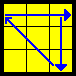x’ R’ D R’ U2 R D’ R’ U2 R2
A'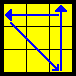x’ R2 U2 R D R’ U2 R D’ R Inverse of A
E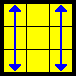(Lw’ U R D’ R’ U’ R D) x’ (Lw’ U’ R D’ R’ U R D) Actually two OLLs in a row

## Alternatives

Name Image Algorithm Comment
E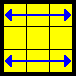z U2 R2 F (R U R’ U’)3 F’ R2 U2 Longer but very easy to remenber.

# Edges only

Name Image Algorithm Comment
U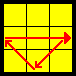R2 U R U R’ U’ R’ U’ R’ U R'
Usym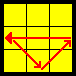L2 U’ L’ U’ L U L U L U’ L Left-handed U perm
H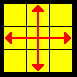M2’ U M2’ U2 M2’ U M2' M2’ with ring finger + middle finger
Z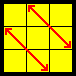M’ U M2’ U M2’ U M’ U2 M2’ [U'] M2’ with ring finger + middle finger

## Alternatives

Name Image Algorithm Comment
HR2 U2’ R U2’ R2’ U2’ R2 U2’ R U2 R2' Better on bigger cubes
Z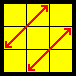R U R’ U R’ U’ R’ U R U’ R’ U’ R2’ U R [U2] Better on bigger cubes

# Two adjacent corners and two edges

Name Image Algorithm Comment
T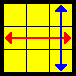(R U R’ U’) R’ F R2 U’ R’ U’ (R U R’ F')
J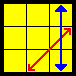(R U R’ F’) (R U R’ U’) R’ F R2 U’ R’ [U'] Same as T but with the last 4 moves now at the beginning
Jsym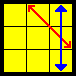L’ R’ U2 R U R’ U2 L U’ R [U]
F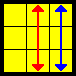R’ U’ F’ (R U R’ U’) R’ F R2 U’ (R’ U’ R U) R’ U R T perm with an (R’ U’ F’) setup, and a cancellation at the end
R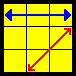(R’ U2 R U2) (R’ F R U R’ U’) (R’ F’ R2) [U']
Rsym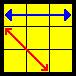(L U2’ L’ U2’) (L F’ L’ U’ L U) (L F L2’) [U] Left-handed R perm

# The Gs

There is only one algorithm here. The second one is the inverse of the first one (after replacing y’RU’R’ with the equivalent yLU’L). The other two are the left-hand equivalents.

Name Image Algorithm Comment
G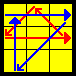(R2 Uw) (R’ U R’ U’ R Uw’) R2’ y (L U’ L') FU-FUR same, FUL adjacent
G'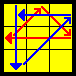y’ (R’ U’ R) y R2’ (Uw R’ U R U’ R) (Uw’ R2') FU-FUR same, FUL opposite
Gsym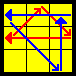(L2’ Uw’) (L U’ L U L’ Uw) L2 y’ (R U’ R') FU-FUL same, FUR adjacent
G’sym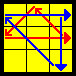y (L U L’) y’ L2’ (Uw’ L U’ L’ U L’) (Uw L2) FU-FUL same, FUR opposite

# Diagonals

Name Image Algorithm Comment
V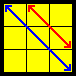(R’ U R’ U’) y (R’ F’ R2 U’) (R’ U R’ F) (R F)
N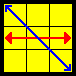(R’ U L’ U2’ R U’ L)2 [U]
Nsym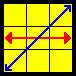(L U’ R U2 L’ U R’)2 [U'] Left-handed N perm
Y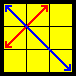F (R U’ R’ U’) (R U R’ F’) (R U R’ U’) (R’ F R F')

## Alternatives

Name Image Algorithm Comment
V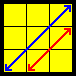z U’ R D (R’ U R U’) z’ (U R’ U’ L) (U2 R U2 R') Better for one-handed solves